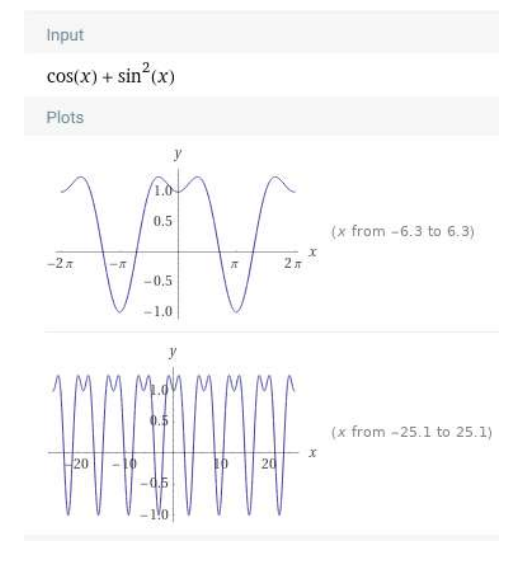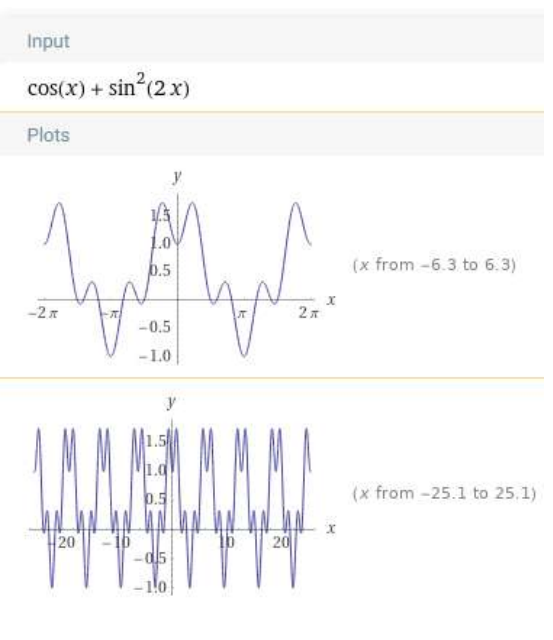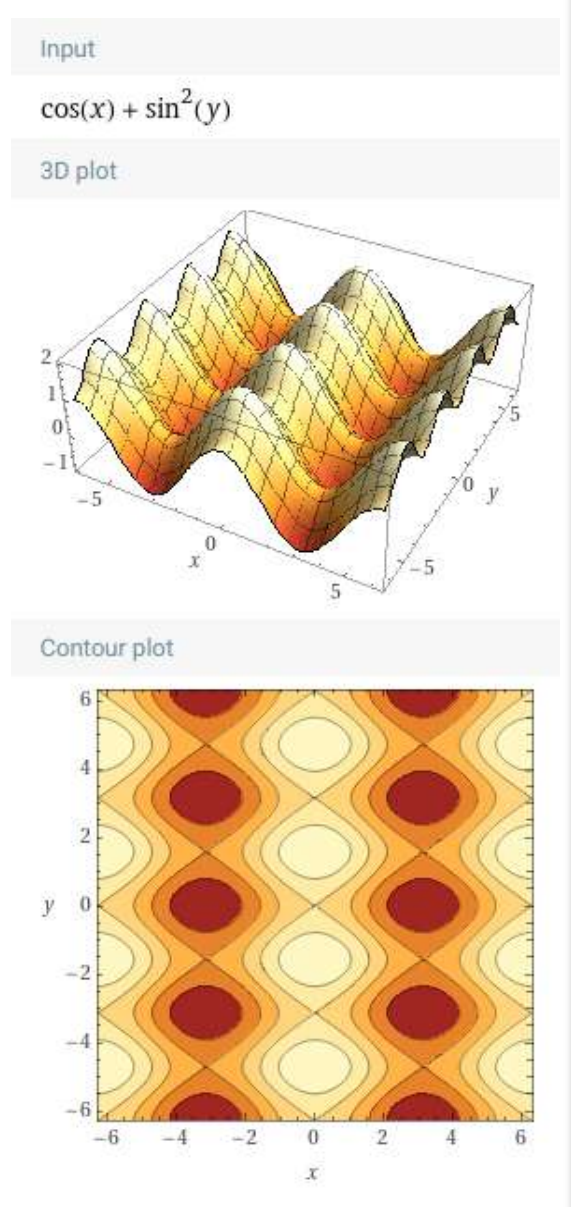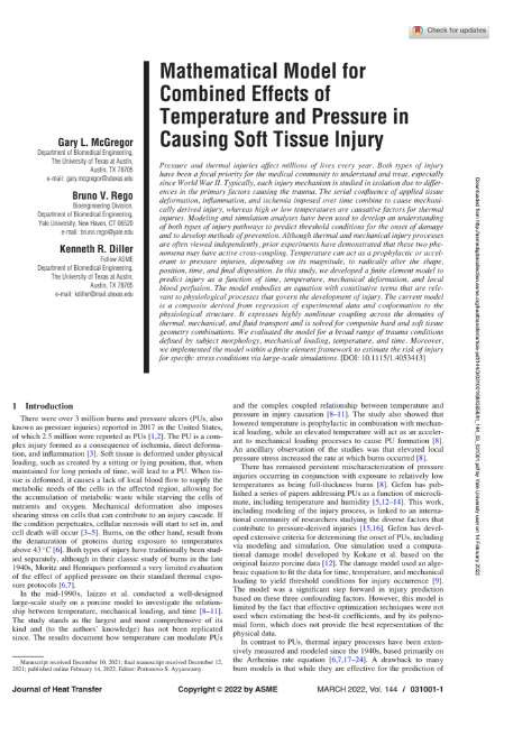#### “An algebra equation should have a physical meaning to it, and have good real world examples…” – Gary McGregor, PhD

Gary McGregor has a a lot to say when it comes to math because he survived dropping out of high school, knowing only the basics of math calculation (adding and subtracting, but not fractions) before he enrolled in Junior College determined to learn all the math he would need to become a mechanical engineer.

Not only did he succeed in that goal, he became an expert higher mathematician and now publishes papers involving complex math.

So how did he get from A to Z?

##### MATH FROM MULTIPLE PERSPECTIVES

Gary was fortunate to learn from a math teacher in junior college who was comfortable allowing students to solve problems in their own way. Gary told me that he thinks he and other dyslexics often prefer to start at the answer and work backwards, instead of imitating a step-by-step sequential process (since coming out of about being dyslexic, he has given advice to others).

##### UNDERSTANDING IS ESSENTIAL

Rote math may be next to impossible for some dyslexic students to learn; so lessons must be directed at helping students understand why and not just how problems are solved, otherwise lessons will not stick.

As an example for how Gary said he would work with mathematical equations and notations until they had meaning, he shared how he used a program called Wolfram Alpha to get a visual sense for what math relationships meant.

If he wanted to understand: f(x) = cos(x) + sin(x)^2See what happens with the equation changed say: f(x) = cos(x) + sin(2x)^2By playing around like this it will help in understanding the math rule they are teaching and what effect they have on manipulating the equations. A simplification but accurate assumption is equations are like machines you put something in and it spits something out. Change the comments of the machine and change the output.

What would a 3D version of the equation look like? f(x) = cos(x) + sin(y)^2From Gary again: “Understanding the behavior and shape helps make math from a bunch of odd words and symbols to something more physical or visual.

It is not demonstrated in this example but I use this type of technique and others similar to learn challenging or new mathematical areas. By physically playing with the equations you can better understand the rules and what they mean, and gain a physical intuition as to how it works.”

If you’re curious about how Gary uses higher math in the work he does now, click on the paper below.Gary reinforced to me his belief that mindset is important to address with math learning. Become interested and curious about the process and play with different variables and relationships and see what will change.

Other tools Gary recommended for math, engineering, and general learning and memory:

Matlab

Matlab GUI a 3d printer to create physical models.

Jim Kwik (memory)

Magnetic Memory

MacGyver Method when stuck or need a different perspective